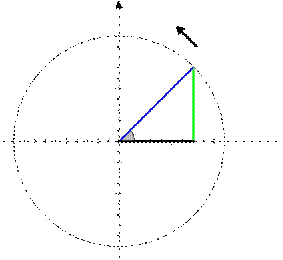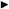3. (a) A projectile fired at an angle? has its horizontal (x) and vertical (y) distance as a function of time, t, given as: x =utcos(?) y =utsin(?) ?gt22 where g is the acceleration due to gravity, and u is the initial velocity of the projectile. At what time does the projectile reaches the highest point in its trajectory? Express your answer in terms of u, g, and? (b) Similarly, determine the maximum height. (c) At what time after launch does the projectile hit the ground? (d) Evaluatedy andd 2y dx dx2 [3 marks] [3 marks] [3 marks] [6 marks] (e) Use that u = 100 m/s and g = 10 m/s2. Make a sketch of the trajectory of the projectile for a launch at an angle with the horizon of?= 30° and in the same sketch, add the trajectory for a launch angle?= 70° (f) Show that the horizontal distance travelled by the projectile can be written as: x =2gu2sin ?cos ? For which value of? does the projectile travels furthest? 4. (a) Let z= 1+ j and 1? j be two complex numbers. Determine the following: (i) z+ w (ii) z? w (iii) zw (iv) z w (b) Express the complex number?4 in modulus-argument form. Hence find all solutions z to the equation z4+4=0and mark them on an Argand diagram. (c) [5 marks] [5 marks] [9 marks] [9 marks] Page 3 of 5 y P( t) R ?t x Fig 3 5 (a) (b) (c) (d) Consider a simple harmonic oscillator whose motion is illustrated in the diagram above. We can describe the motion in complex form as P(t)= Rej?t. Determine P(t)= Rej?t in rectangular form for R= 2 and (i)?t= ? (ii)?t= ? (iii)?t=? 4 2 Solve the following linear system of equations: 10x + y ? 5z = 18 ?20x +3y + 20z =14 5x + 3 y + 5z = 9 Determine AB if possible for the following matrices: 1 1 (a) B= (1 2 3 4) (b) A= B=(5 6) A= , 2 , 7 3 (c) A=(1 3 (d) A = 3 B=(1 2) 2) , B= 4 4 , ?1 0 0 0 ?1 0 . DetermineA 2013 . LetA= 0 0 ?1 1 1 0 0 LetA=(1 2 3 ), B = 2 0 2 0 . Evaluate ADB. and D= 3 0 0 3 [7 marks] 10 marks 8 marks 2 marks 5 marks Page 4 of 5 (a) Evaluate the following: ?24 x3dx ?42 x3dx 6 marks (b) Let f( x)=?03 ex2 dx Determine the derivative f?( x) . 4 marks (i) Show that dxd sin(x)ln(x)= cos( x) ln( x)+sinx(x) 4 marks Find ? cos( x) ln( x)+ sin( x)dx x 3 marks

3. (a) A projectile fired at an angle? has its horizontal (x) and vertical (y) distance as a function of time, t, given as:

x =utcos(?)

y =utsin(?) ?gt22

where g is the acceleration due to gravity, and u is the initial velocity of the projectile.

At what time does the projectile reaches the highest point in its trajectory? Express your answer in terms of u, g, and?

(b) Similarly, determine the maximum height.

(c) At what time after launch does the projectile hit the ground?

(d) Evaluatedy andd 2ydx dx2

[3 marks] [3 marks] [3 marks]

[6 marks]

(e) Use that u = 100 m/s and g = 10 m/s2. Make a sketch of the trajectory of the projectile for a launch at an angle with the horizon of?= 30° and in the

same sketch, add the trajectory for a launch angle?= 70°

(f) Show that the horizontal distance travelled by the projectile can be written as:

x =2gu2sin ?cos ?

For which value of? does the projectile travels furthest?

 4. (a) Let z= 1+ j and 1? j be two complex numbers. Determine the following: (i) z+ w (ii) z? w (iii) zw (iv) z w

(b) Express the complex number?4 in modulus-argument form. Hence find all solutions z to the equation

z4+4=0and mark them on an Argand diagram.

(c)

[5 marks]

[5 marks]

[9 marks]

[9 marks]

Page 3 of 5y

P( t)

R

?t

xFig 3

5 (a)

(b)

(c)

(d)

Consider a simple harmonic oscillator whose motion is illustrated in the diagram above. We can describe the motion in complex form as

P(t)= Rej?t.

Determine P(t)= Rej?t in rectangular form for R= 2 and

 (i)?t= ? (ii)?t= ? (iii)?t=? 4 2
 Solve the following linear system of equations: 10x + y ? 5z = 18 ?20x +3y + 20z =14 5x + 3 y + 5z = 9

Determine AB if possible for the following matrices:

 1 1 (a) B= (1 2 3 4) (b) A= B=(5 6) A= , 2 , 7 3 (c) A=(1 3 (d) A = 3 B=(1 2) 2) , B= 4 4 , ?1 0 0 0 ?1 0 . DetermineA 2013 . LetA= 0 0 ?1 1 1 0 0 LetA=(1 2 3 ), B = 2 0 2 0 . Evaluate ADB. and D= 3 0 0 3

[7 marks]

10 marks

8 marks

2 marks

5 marks

Page 4 of 5

1. (a) Evaluate the following:
1. ?24 x3dx
2. ?42 x3dx

6marks

(b) Let f( x)=?03 ex2 dx Determine the derivative f?( x) .

4 marks

1. (i) Show that

dxd sin(x)ln(x)= cos( x) ln( x)+sinx(x)4 marks

1. Find
 ? cos( x) ln( x)+ sin( x)dx x

3 marks